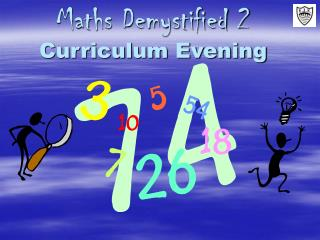Download PresentationMaths Demystified 2 Curriculum Evening

# Maths Demystified 2 Curriculum Evening

Download Presentation## Maths Demystified 2 Curriculum Evening

- - - - - - - - - - - - - - - - - - - - - - - - - - - E N D - - - - - - - - - - - - - - - - - - - - - - - - - - -
##### Presentation Transcript

1. Maths Demystified 2 Curriculum Evening

2. What’s coming up: • Why have a Maths Evening? • How children progress in Maths • The 4 rules: how are they taught now at FJS? • Mental strategies • Written strategies • Importance of problem solving • How can you help your child at home? • Information on further workshops for parents Working together with your child • Refreshments

3. Structure of the Daily Mathematics Lesson • Mental and Oral Starter • Main Input • Group work • Plenary Sharing and articulation of strategies is vital, also representing the strategies visually

4. Progression in Maths • Children start by learning mental methods of calculation before moving onto informal jottings (such as the number line). • When these are understood the children then learn the formal layout of calculations. • Moving ahead too quickly or missing a step can lead to children becoming confused and insecure.

5. Addition • Children are taught a range of strategies for adding numbers in their heads. • They learn ‘number bonds’ to 10 and 100 • They learn how to count on for small differences. • They also learn how to add numbers that are close to a multiple of ten and then adjust such as 49 + 23 = 50 + 23 – 1= 72

6. Addition – Written method • On the way to learning the formal written method, children learn column addition, starting with the least significant digit.

7. Subtraction – Mental Strategies • Counting on or back e.g. in 5s, 10s, 100s • Counting on or back to solve problems e.g. 86-30 • Partitioning (splitting the number up : one number or both) • Compensating • Subtracting time (non-metric) • Selecting the right method

8. Subtraction –written methods • Number line • Written representation of the number line (vertical) • Traditional formal written method

9. Multiplication • Essential that children learn their tables (and related division facts). • The booklet gives examples of games you can play at home to reinforce.

10. Multiplication • Ideally… • By the end of year 3 children should know their 2, 5 and 10 times tables. • By the end of year 4 children should know their 2, 3, 4, 5, and 10 times tables. • By the end of year 5 children should know all their tables to x10. • By the end of year 6 children should be able to give the right answer before the question is asked.

11. Multiplication – written method • One of the written methods the children learn is the grid method. • This builds on their knowledge of place value and of multiplying smaller numbers.

12. Division – mental methods • Knowledge of tables – backwards and inside out • 4x9=36, 9x4=36, 36÷9=4, 36÷4=9 • Use of arrays • Dividing by 10, 100, 1000 • Halving (also ÷4 is halving and halving again) • Finding fractions, decimals and percentages e.g. 1/3 of 27, 25% of £10, 0.5 x 12

13. Division – the importance of context68 ÷ 8 • Harry has 68 toffees he shares them between 8 friends. How many does each friend get and how many are left over? • A 68cm piece of ribbon is cut into 8 equal pieces. How long is each piece? • 68 scouts go on a weekend camping trip. Each tent takes 8 scouts. How many tents will they need to bring? • Pencils come in boxes of 8. How many full boxes can you make from 68 pencils?

14. Division –written methods • Repeated subtraction or ‘chunking’

15. Problem solving Emily uses 3 silver coins to pay for an orange. The orange costs 67p. How much change does she get? The answer is 4.7cm. What is the problem? • Puts Maths in a real context • Allows children to investigate different ways of solving a problem • There is often not just one ‘right’ answer but many possible answers

16. How can you help at home? • Have a positive view of Maths with your child • Ask them to teach you what they learnt today • Involve children with weighing and measuring in real contexts e.g. cooking • Give them the responsibility of handling money • Play maths games or games that involve Maths e.g. Yahzee, Monopoly, Card Games e.g. Cribbage, 21 or bust etc. • Practise the mental arithmetic basics a little and often e.g. adding car number plates • Using time e.g. You’ve got 40 minutes on your PS2 - what time do you need to stop playing? • Maths is all around – make the most of natural opportunities

17. Free Workshops For Parents • Roz Bradbury

18. Workshops • Whiteboard warm up • Working together with your child on an activity • Refreshments in the Hall afterwards